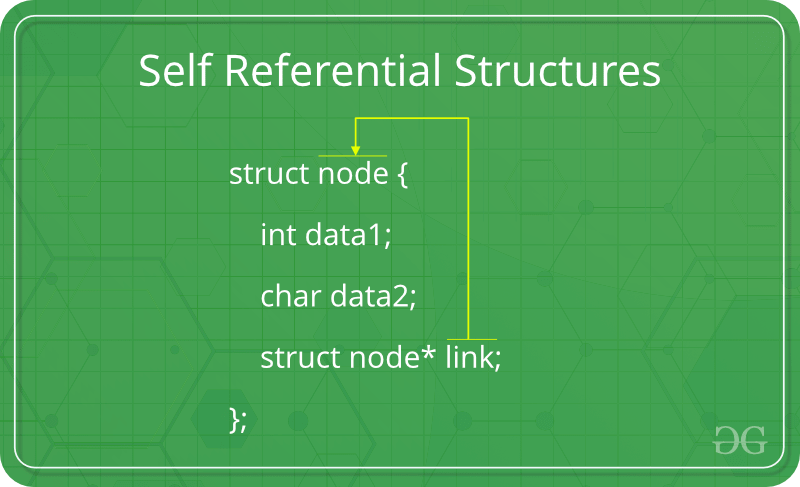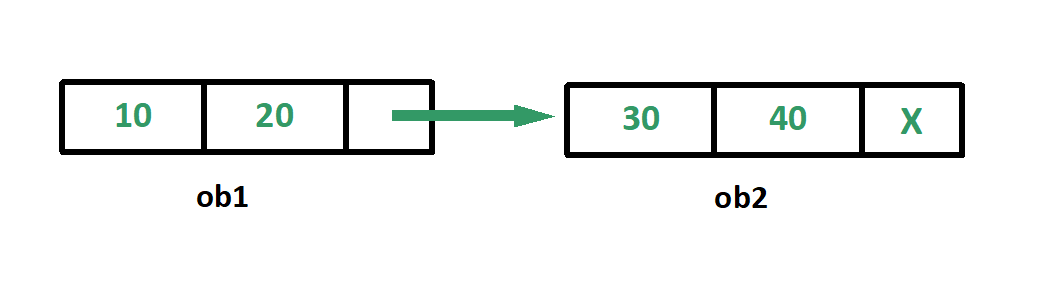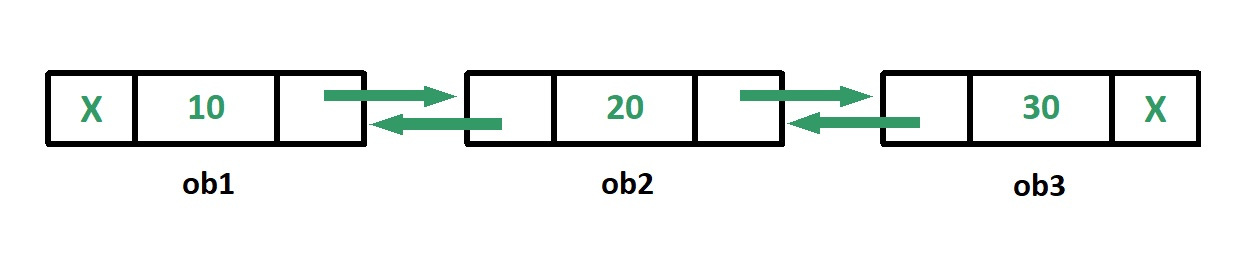# Self Referential Structures

• Difficulty Level : Medium
• Last Updated : 03 Nov, 2021

Self Referential structures are those structures that have one or more pointers which point to the same type of structure, as their member.In other words, structures pointing to the same type of structures are self-referential in nature.
Example:

## CPP

 `struct` `node {``    ``int` `data1;``    ``char` `data2;``    ``struct` `node* link;``};` `int` `main()``{``    ``struct` `node ob;``    ``return` `0;``}`

## Python3

 `class` `node:``    ``def` `__init__(``self``):``        ``self``.data1``=``0``        ``self``.data2``=``''``        ``self``.link``=``None` `if` `__name__ ``=``=` `'__main__'``:``    ``ob``=``node()`

In the above example ‘link’ is a pointer to a structure of type ‘node’. Hence, the structure ‘node’ is a self-referential structure with ‘link’ as the referencing pointer.
An important point to consider is that the pointer should be initialized properly before accessing, as by default it contains garbage value.
Types of Self Referential Structures

1. Self Referential Structure with Single Link
2. Self Referential Structure with Multiple Links

Self Referential Structure with Single Link: These structures can have only one self-pointer as their member. The following example will show us how to connect the objects of a self-referential structure with the single link and access the corresponding data members. The connection formed is shown in the following figure.## CPP

 `#include ` `struct` `node {``    ``int` `data1;``    ``char` `data2;``    ``struct` `node* link;``};` `int` `main()``{``    ``struct` `node ob1; ``// Node1` `    ``// Initialization``    ``ob1.link = NULL;``    ``ob1.data1 = 10;``    ``ob1.data2 = 20;` `    ``struct` `node ob2; ``// Node2` `    ``// Initialization``    ``ob2.link = NULL;``    ``ob2.data1 = 30;``    ``ob2.data2 = 40;` `    ``// Linking ob1 and ob2``    ``ob1.link = &ob2;` `    ``// Accessing data members of  ob2 using ob1``    ``printf``(``"%d"``, ob1.link->data1);``    ``printf``(``"\n%d"``, ob1.link->data2);``    ``return` `0;``}`

## Python3

 `class` `node:``    ``def` `__init__(``self``):``        ``self``.data1``=``0``        ``self``.data2``=``0``        ``self``.link``=``None` `if` `__name__ ``=``=` `'__main__'``:``    ``ob1``=``node() ``# Node1` `    ``# Initialization``    ``ob1.link ``=` `None``    ``ob1.data1 ``=` `10``    ``ob1.data2 ``=` `20` `    ``ob2``=``node() ``# Node2` `    ``# Initialization``    ``ob2.link ``=` `None``    ``ob2.data1 ``=` `30``    ``ob2.data2 ``=` `40` `    ``# Linking ob1 and ob2``    ``ob1.link ``=` `ob2` `    ``# Accessing data members of  ob2 using ob1``    ``print``(ob1.link.data1)``    ``print``(ob1.link.data2)`
Output:
```30
40```

Self Referential Structure with Multiple Links: Self referential structures with multiple links can have more than one self-pointers. Many complicated data structures can be easily constructed using these structures. Such structures can easily connect to more than one nodes at a time. The following example shows one such structure with more than one links.
The connections made in the above example can be understood using the following figure.## CPP

 `#include ` `struct` `node {``    ``int` `data;``    ``struct` `node* prev_link;``    ``struct` `node* next_link;``};` `int` `main()``{``    ``struct` `node ob1; ``// Node1` `    ``// Initialization``    ``ob1.prev_link = NULL;``    ``ob1.next_link = NULL;``    ``ob1.data = 10;` `    ``struct` `node ob2; ``// Node2` `    ``// Initialization``    ``ob2.prev_link = NULL;``    ``ob2.next_link = NULL;``    ``ob2.data = 20;` `    ``struct` `node ob3; ``// Node3` `    ``// Initialization``    ``ob3.prev_link = NULL;``    ``ob3.next_link = NULL;``    ``ob3.data = 30;` `    ``// Forward links``    ``ob1.next_link = &ob2;``    ``ob2.next_link = &ob3;` `    ``// Backward links``    ``ob2.prev_link = &ob1;``    ``ob3.prev_link = &ob2;` `    ``// Accessing  data of ob1, ob2 and ob3 by ob1``    ``printf``(``"%d\t"``, ob1.data);``    ``printf``(``"%d\t"``, ob1.next_link->data);``    ``printf``(``"%d\n"``, ob1.next_link->next_link->data);` `    ``// Accessing data of ob1, ob2 and ob3 by ob2``    ``printf``(``"%d\t"``, ob2.prev_link->data);``    ``printf``(``"%d\t"``, ob2.data);``    ``printf``(``"%d\n"``, ob2.next_link->data);` `    ``// Accessing data of ob1, ob2 and ob3 by ob3``    ``printf``(``"%d\t"``, ob3.prev_link->prev_link->data);``    ``printf``(``"%d\t"``, ob3.prev_link->data);``    ``printf``(``"%d"``, ob3.data);``    ``return` `0;``}`

## Python3

 `class` `node:``    ``def` `__init__(``self``):``        ``self``.data``=``0``        ``self``.prev_link``=``None``        ``self``.next_link``=``None` `if` `__name__ ``=``=` `'__main__'``:``    ``ob1``=``node() ``#Node1` `    ``# Initialization``    ``ob1.prev_link ``=` `None``    ``ob1.next_link ``=` `None``    ``ob1.data ``=` `10` `    ``ob2``=``node() ``#Node2` `    ``# Initialization``    ``ob2.prev_link ``=` `None``    ``ob2.next_link ``=` `None``    ``ob2.data ``=` `20` `    ``ob3``=``node() ``# Node3` `    ``# Initialization``    ``ob3.prev_link ``=` `None``    ``ob3.next_link ``=` `None``    ``ob3.data ``=` `30` `    ``# Forward links``    ``ob1.next_link ``=` `ob2``    ``ob2.next_link ``=` `ob3` `    ``# Backward links``    ``ob2.prev_link ``=` `ob1``    ``ob3.prev_link ``=` `ob2` `    ``# Accessing  data of ob1, ob2 and ob3 by ob1``    ``print``(ob1.data,end``=``'\t'``)``    ``print``(ob1.next_link.data,end``=``'\t'``)``    ``print``(ob1.next_link.next_link.data)` `    ``# Accessing data of ob1, ob2 and ob3 by ob2``    ``print``(ob2.prev_link.data,end``=``'\t'``)``    ``print``(ob2.data,end``=``'\t'``)``    ``print``(ob2.next_link.data)` `    ``# Accessing data of ob1, ob2 and ob3 by ob3``    ``print``(ob3.prev_link.prev_link.data,end``=``'\t'``)``    ``print``(ob3.prev_link.data,end``=``'\t'``)``    ``print``(ob3.data)`
Output:
```10    20    30
10    20    30
10    20    30```

In the above example we can see that ‘ob1’, ‘ob2’ and ‘ob3’ are three objects of the self referential structure ‘node’. And they are connected using their links in such a way that any of them can easily access each other’s data. This is the beauty of the self referential structures. The connections can be manipulated according to the requirements of the programmer.
Applications:
Self referential structures are very useful in creation of other complex data structures like:

My Personal Notes arrow_drop_up